# Difference between revisions of "Testpage1"

Tidal asymmetry and tidal basin morphodynamics

This article describes the physical processes responsible for tidal wave deformation in shallow coastal inlet systems – tidal lagoons and estuaries. A qualitative discussion is given of the mutual interaction between tidal asymmetry generation and morphological development of these systems, which is generally referred to by the term self-organizing morphodynamics. This article is largely based on the book Dynamics of Coastal Systems .

## Tidal wave deformation in shallow water

Ocean tidal waves are distorted when they propagate into shallow coastal waters. Here the term tidal wave distortion is to be understood as a systematic (long-term averaged) difference between the periods of rising and falling tide, also called tidal asymmetry (Fig.1). We will use the term positive duration asymmetry for tides with a longer fall and shorter rise and negative duration asymmetry for tides with a shorter fall and longer rise. Nonlinear hydrodynamic processes that are insignificant in the deep ocean become important when the tidal range is larger than a small fraction of the mean water depth. In some cases these nonlinear processes can lead to the development of a tidal bore: a sudden elevation of the water level propagating upstream an estuary or a tidal river. In the following we shortly review the major nonlinear processes involved in initiating shallow-water tidal distortion.

However, it must be noted before that linear ocean tides are not fully symmetric, due to the superposition of certain tidal components with mutually related frequencies (for example, the sum of the frequencies of the K1 and O1 tides is equal to the frequency of the M2 tide). Although this was known for a long time (Doodson, 1921), it has received renewed attention more recently due to the observations and analyses of Hoitink et al. (2003) and several other studies since (Nidzieko, 2010; Zhang, 2018 ). A world map showing ocean regions with asymmetric tides was published by Song et al. (2011), based on tidal constants derived from the TPXO7-ATLAS (http://volkov.oce.orst.edu/tides/atlas.html). Asymmetric tides (with either positive or negative duration asymmetry) occur mainly in regions where tides have a mixed character, with comparable magnitudes of diurnal and semidiurnal tidal components.

Although the asymmetry of ocean tides can be significant, tidal asymmetry can become much stronger due to the generation of shallow-water overtides. These overtides are important in regions with a large semidiurnal tidal range (dominated by M2). We focus here on asymmetry generated by the interaction of tides with topographic characteristics. Most studies of overtide generation consider estuaries and tidal rivers with simple one-dimensional geometries. In these studies, the influence of channel curvature, secondary channels or local channel constrictions on tidal asymmetry are ignored. Asymmetries related to density gradients or wind-driven currents are also left out of consideration.

Tides result from the response of the ocean water bodies to the attractive gravitational forces by sun and moon. Tidal motion in the oceans can be described by a limited number of sinusoidal components, because earth's rotation and the relative movements of sun and moon have a cyclical character, see the article Ocean and shelf tides. The semidiurnal lunar tide (M2) is usually the dominant component, in which case the ocean tide can be described fairly accurately with a single sine function.

However, it is well known that this simple description is not valid for shallow coastal areas. The tidal wave is distorted as it propagates from the ocean to coastal areas where the tidal amplitude is no longer negligibly small compared to the average water depth. Negligibly small means: a tidal amplitude that is no greater than a few percent of the water depth. In some coastal areas, particularly in estuaries and tidal lagoons, the tidal amplitude is just a few times smaller than the average water depth or even of similar magnitude.

In shallow coastal areas, the distortion of the tide can be so strong that the durations of rising tide and falling tide become very different and that a large difference arises between the peak flow velocities of flood and ebb. Often the duration of rising tide is much shorter than the duration of falling tide. This is illustrated in Fig. 1 for the Hooghly estuary. In the most extreme case, the duration of tidal rise becomes so short that a hydraulic jump develops at the front of the tidal wave. The front of the tidal wave appears as a propagating wall of water, a so-called tidal bore.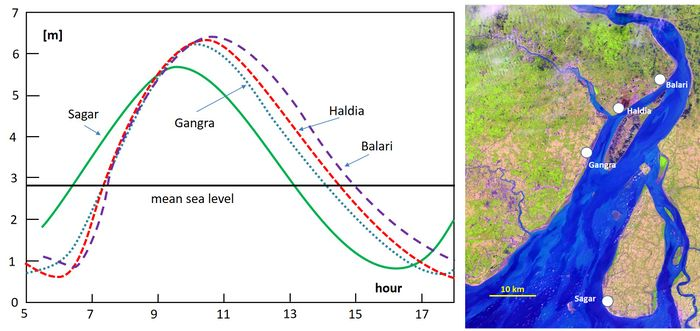Figure 1: Tide curves in the Hooghly estuary observed during a high springtide (19/9/2009) at different tide gauges along the estuary. Data from Banerjee et al. (2015)

## Tidal wave deformation in the absence of friction

The distortion of the tide described above is related to a difference in propagation speed of the high-water crest of the tidal wave and the low-water trough. This difference already occurs when the water depth below the wave crest is slightly greater than the water depth below the wave trough. This can be easily demonstrated for a tidal wave that propagates in $x$-direction with time $t$ in deep water with little loss of friction. The tidal equations (momentum balance and mass balance) can in this case be simplified to

$\Large\frac{\partial u}{\partial t}\normalsize + u \Large \frac{\partial u}{\partial x}\normalsize + g \Large \frac{\partial \zeta}{\partial x}\normalsize =0 , \quad \quad (1)$

$\Large \frac{\partial \zeta}{\partial t}\normalsize + h \Large \frac{\partial u}{\partial x}\normalsize + \Large \frac{\partial u \zeta }{\partial x}\normalsize =0 , \quad \quad (2)$

where $\zeta$ is the tidal wave surface elevation, $u$ is the depth-averaged tidal velocity, $h$ is the mean water depth and $g$ the gravitational acceleration. The equations are linear except for the second term in Eq. (1) and the last term in Eq. (2). These non-linear terms are much smaller in the present case than the linear terms.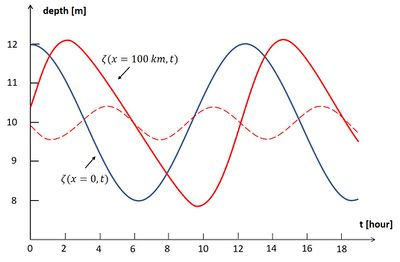Figure 2: Deformation of a frictionless propagating wave, corresponding to Eqs. (1) and (2). The blue curve is the sinusoidal tidal wave at $\small x=0, \zeta(x=0,t) = a \cos \omega t , \; a = 2 m$. The red curve is the distorted tidal wave after travelling a distance of $\small x=$ 100 km without friction in a channel of 10 m mean depth, $\small \zeta(x,t) = \zeta^{(1)} + \zeta^{(2)}$, with $\small \zeta^{(1)} = a \cos(\omega t - kx) , \; \zeta^{(2)} = \frac{3 a^2}{4h} kx \sin(2 \omega t – 2kx)$. The dotted red line is $\small \zeta^{(2)}$. The red curve displays a shorter tidal rise and a longer tidal fall: the tidal wave crest has propagated faster than the tidal wave trough.

The equations can therefore be solved to a first approximation by substituting the solution $\zeta^{(1)}$ of the linear equations into the nonlinear terms. The solution of the resulting linearized equations introduces a small M4 tidal component $\zeta^{(2)}$ with twice the M2-tidal frequency. This M4 component modifies the duration of rising water and falling water, because the propagation speed $c^+$ of the high-water wave crest is higher than the propagation speed $c^-$ of the low-water wave trough:

$c^{\pm}=(1 \pm \Large \frac{3a}{2h}\normalsize) \; \sqrt{gh} , \quad \quad(3)$

where $a$ is the amplitude of the M2 tide. The resulting tidal distortion is illustrated in Fig. 2; a derivation is given in the appendix. This equation suggests that after some time the high-water wave crest overtakes the low-water wave trough. However, this can only happen when the amplitude of the M4 is of the same order of magnitude as the M2 tide, which violates the assumption underlying the linearization of the tidal equations.

The approximate validity of the result (3) is restricted to the zone where M4 is much smaller than M2. In spite of the restricted validity of Eq. (3) to large water depths and small tidal amplitude, the following general rule remains valid: the difference in propagation speed between high water and low water increases when the ratio $a/h$ increases. Numerical simulations with fully nonlinear mathematical models show that tidal distortion, with decreasing tidal rise period, occurs when the value of the parameter $a/h$ remains sufficiently large when the tidal wave propagates over a shallow seabed . In this case, the tidal wave transforms into a tidal bore when the propagation distance over the shallow seabed is sufficiently large.

## Tidal wave deformation in the presence of friction and intertidal areas

Tidal asymmetry develops during up-channel propagation into a shallow tidal basin. Pronounced tidal asymmetry (possibly leading to tidal bore formation) only occurs if during propagation a sufficiently large ratio $a/h$ is maintained. In many cases this condition is not met, because the tidal amplitude decreases during propagation. The two main reasons for decrease of the tidal amplitude are: (1) tidal wave damping by friction and (2) lateral spreading of the flood tidal wave. These two nonlinear processes also influence the propagation of the high-water wave crest and the low-water wave trough. This can be demonstrated in the same way as previously, assuming that the nonlinear terms in the equations are much smaller than the linear terms.

We consider a straight prismatic tidal channel with mean depth $h$ , channel width $b_C$ and lateral flood storage zones of width $b_S= b_{S0} \; ( 1 + \zeta / a)$, where $\zeta / a$ is the ratio of tidal surface elevation and tidal amplitude, see Fig. 3 . When frictional effects are much stronger than inertial effects, the one-dimensional cross-section-averaged tidal equations for small values of $a/h$ can be simplified to

$g \Large \frac{\partial \zeta}{\partial x}\normalsize + r \Large \frac{u}{h+\zeta}\normalsize \approx g\Large \frac{\partial \zeta}{\partial x}\normalsize + r \Large \frac{u}{h}\normalsize – r \Large \frac{u \zeta}{h^2}\normalsize =0 , \quad \quad (4)$

$(b_C+ b_S) \Large \frac{\partial \zeta}{\partial t} \normalsize + \Large \frac{\partial (u b_C(h+\zeta)) }{\partial x} \normalsize = (b_C+ b_{S0}) \Large \frac{\partial \zeta}{\partial t} \normalsize + b_{S0} \Large \frac{\zeta}{a} \frac{\partial \zeta}{\partial t} \normalsize + h b_C \Large \frac{\partial u}{\partial x}\normalsize + b_C \Large \frac{\partial (\zeta u)}{\partial x}\normalsize =0 . \quad \quad (5)$

The friction term in Eq. (4) has been linearized; $r$ is the associated friction coefficient (dimension [m/s]) that relates momentum dissipation at the channel bed to the depth-averaged current velocity. Its value typically ranges between 0.001 - 0.004 m/s . The precise value of the friction coefficient for estuarine flow is generally not well known as it is influenced by many factors such as density stratification (lower friction), small bed forms (higher friction) and fluid mud layers (lower friction). The friction coefficient may even be different for ebb and flood because of differences in salinity stratification. Apart from salinity stratification effects, there is no significant influence of river flow on bed friction in most estuaries. The linearization of the friction term is often a minor approximation compared to the uncertainty in the value of the friction coefficient. Neglect of the inertial term $\partial u / \partial t$ in the momentum equation is a valid approximation if $r / h \omega \lt \lt 1$. In shallow estuaries, $h \le 5 m$, the friction term is on average a few times larger than the inertial term.

In estuaries where friction dominates inertia (assumption underlying the derivation of equation (4)) the tide does not travel as a propagating wave, but rather advances into the estuary through a diffusion-type process, as described by Blondeaux (1978) for the Saint Lawrence Estuary . The tidal wave crest does not coincide with the time of high water, but lags behind. The same applies to low water.

The nonlinear terms in the tidal equations are much smaller than the linear terms if $b_S \lt \lt b_C$ and $a/h \lt \lt 1$. In this case a first order solution $\zeta^{(1)}$ can be found by substituting the solution of the linear equations in the nonlinear terms. The nonlinear terms then generate a small M4 tidal component $\zeta^{(2)}$, which affects the duration of tidal rise and tidal fall. This is because the propagation speed $c^+$ of the high-water wave crest differs from the propagation speed $c^-$ of the low-water wave trough (see the appendix):

$c^{\pm} \approx [1 \pm (2 - \sqrt{2}) \Large\frac{a}{h}\normalsize \mp \Large\frac{b_{S0}}{2b_C}\normalsize ] \; \sqrt{gh} \; \sqrt{ \Large\frac{2 \omega h}{r} \frac{b_C}{b_C +b_{S0}}\normalsize} , \quad \quad (6)$

where $\omega$ is the M2 radial frequency. This expression shows that the tidal propagation speed is reduced for increasing values of the nondimensional friction coefficient $r / (h \omega)$. As in the previous example, the propagation speed of the high-water (HW) wave crest $c^+$ increases and the propagation speed of the low-water (LW) wave trough $c^-$ decreases with increasing values of $a/h$. The propagation of low water is more delayed than the propagation of high water, due to stronger friction at smaller water depths. The period during which the tide rises is thus shortened with respect to the duration of falling tide. However, the opposite occurs for increasing values of the relative flood storage width $b_{S0}/b_C$. The HW propagation speed decreases relative to the LW propagation and the period during which the tide rises is thus lengthened with respect to the duration of falling tide. This can be understood by noting that the lateral expansion of the tidal flood wave over the intertidal area reduces its propagation speed and thus delays the time of HW in the upper-channel zone. Hence, shallowness of the channel (large $a/h$) and extension of the intertidal area (large $b_{S0}/b_C$) have counteracting effects on tidal wave distortion.

The most simple geometry is an infinite prismatic channel. The mean (tide-averaged) channel depth is called $h$ (constant), the tidal elevation is called $\zeta(x,t)$, the total instantaneous water depth $D(x,t)=h+\zeta(x,t)$ and the cross-sectionally averaged velocity $u(x,t)$. The longitudinal coordinate is $x$ and the time $t$.

First we consider tidal propagation in the absence of friction. The tidal equations for mass and momentum balance then read

$\Large\frac{\partial \zeta}{\partial t}\normalsize +\Large\frac{\partial(Du)}{\partial x}\normalsize=0 , \quad \quad (1)$

$\Large\frac{\partial u}{\partial t}\normalsize + u \Large\frac{\partial u}{\partial x} \normalsize + g \Large\frac{\partial \zeta}{\partial x}\normalsize = 0 , \quad \quad (2)$

where $g$ is the gravitational acceleration. Overtides are due to the nonlinear terms $\partial(\zeta u) / \partial x$ and $u \partial u / \partial x$. The quadratic nature of these terms implies that the first and most important overtide (M4) has twice the frequency of the M2 tide. The tidal equations (1,2) can be cast in the form of a characteristic equation that describes the propagation of the tidal wave $\zeta(x,t)$ in positive x-direction,

$\Large\frac{d}{dt}\normalsize \zeta(x,t) = 0 , \quad \Large\frac{dx}{dt}\normalsize = 3 c + u_0 – 2 c_0 , \quad \quad (3)$

where $c=\sqrt{gD(x,t)} , \; c_0= \sqrt{gD(0,0)} , \; u_0=u(0,0) .$. A derivation is given in the appendix. We choose $x=0, t=0$ at high water (HW) and assume that the relative tidal amplitude $a/h$ during the time $t$ remains small ($a/h \lt \lt 1$). Then we have $D(0,0) \approx h+a , \; u(0,0) \approx a\sqrt{g/h}$ as first-order solution of Eqs. (1) and (2). According to Eq. (3), HW thus propagates with velocity

$c^+ = 3 c + u_0 – 2 c_0 = 3 \sqrt{g(h+a)} + a \sqrt{g/h} – 2 \sqrt{g(h+a)} \approx \sqrt{gh} (1 + \Large\frac{3a}{2h}\normalsize) . \quad \quad (4)$

In the same way one finds for the propagation velocity of the low-water (LW) location $c^- \approx \sqrt{gh} (1 – 3a /2h)$. As HW propagates faster than LW, the tidal wave front will steepen progressively; the duration of rising tide shortens while the duration of falling tide is lengthened. This positive tidal asymmetry increases with the relative tidal amplitude $a/h$ as a consequence of the nonlinear terms $\partial(\zeta u) / \partial x$ and $u \partial u / \partial x$ in the tidal equations.

The tidal equations for a prismatic channel do not well represent tidal propagation in estuaries because of neglect of the friction term. The equations (1,2) are more representative for the along-shore propagation of a tidal Kelvin wave, far away from hard boundaries where the tidal wave can reflect or from hydrodynamic boundaries where the Kelvin wave meets other tidal wave systems. An increasing tidal asymmetry develops in situations where the coastal zone is shallow and the tidal amplitude is large. The increasing tidal asymmetry along the coast of Normandy (France can be understood this way (Fig. 2), as well as the increasing tidal asymmetry along the North Sea coast of Holland (Dronkers, 2017). The tidal wave that enters estuaries situated along such coasts (the Seine, for instance) exhibits already significant positive-duration tidal asymmetry.Figure 2. Increasing positive asymmetry ($\Delta_{FR}$ is the difference of the durations of tidal fall and tidal rise) of the tidal wave propagating along the coast of Normandy (France). Tide gauge data of 29 September 2015.

The general tidal equations for estuaries are too complex for an analytic treatment in which tidal asymmetry is explicitly related to the nonlinear terms. The equations can be solved when the nonlinear terms are linearized by assuming that their contribution is relatively small (Lanzoni and Seminara, 1998). Insight in the role of the most important nonlinear terms can be gained when simplifications are made. The corresponding estuarine geometry is shown in Fig. 3. The mean water depth $h$ is uniform throughout the estuary; the channel width $B$ converges exponentially and the surface width $B_S$ increases linearly from the LW level up to the HW level,

$B = b_c e^{-x/L_b} , \; B_S =B + b_S e^{-x/L_b} , \; b_S = \Delta b (1 + \zeta / h) .$

The instantaneous local depth is $D(x,t)=h+\zeta(x,t)$ and the cross-sectional averaged tidal velocity is $u(x,t)$. Density gradients are left out of consideration. Tidal propagation along the estuary can then be described by the mass and momentum balance equations

$B_S \Large\frac{\partial \zeta}{\partial t}+\frac{\partial}{\partial x}\normalsize (BDu) = 0 , \quad \quad (5)$

$\Large\frac{\partial u}{\partial t}\normalsize + u \Large\frac{\partial u}{\partial x} \normalsize + g \Large\frac{\partial \zeta}{\partial x}\normalsize + F = 0 , \quad \quad (6)$

In the last equation $F$ stands for the frictional momentum dissipation which is usually represented by a quadratic expression of the form $c_D |u|u / D .$ Although it appears from detailed measurements that this expression is a rough approximation – the friction coefficient $c_D$ is found to be variable both temporally and spatially (Lewis and Lewis, 1987; Stacey and Ralston, 2005; Lefebvre et al., 2012) – we consider here a further approximation assuming that quadratic dependence on $u$ can be ignored, $F = r u /D ,$ where r is a constant friction coefficient with dimensions [m/s].

In many shallow estuaries with strong tides the friction term is substantially larger than the inertial terms $\partial u / \partial x$ and $u \partial u / \partial x$ and. Such strong friction would cause strong damping of the tidal amplitude along the estuary. In strongly converging estuaries, however, the tidal amplitude appears to be fairly uniform along the estuary (Friedrichs and Aubrey, 1994; Prandle, 2004; Savenije, 2012). Tidal amplification by the funneling effect approximately cancels tidal damping due to friction. In a strongly, exponentially converging estuary the tide is propagating upstream with a phase difference of approximately 90° between tidal elevation and tidal velocity (Jay, 1991). In some estuaries the funneling effect even produces an increase of the tidal amplitude in the strongly converging part of the estuary; examples are the Hooghly, Scheldt, Humber, Gironde-Garonne. This means that the funneling term in Eq. (5), $Du \partial B / \partial x$, is substantially larger than the term $B \partial (Du) / \partial x$. Retaining only the largest terms in equations (5) and (6), the two equations can be combined by eliminating $u$. This yields the simple characteristic equation

$\Large\frac{d}{dt}\normalsize \zeta(x,t) = 0 , \quad \Large\frac{dx}{dt}\normalsize = c = \Large\frac{g b_C D^2}{r b_S L_b}\normalsize , \quad \quad (7)$

If the relative tidal amplitude $a/h$ and the relative intertidal area $\Delta b / b_C$ are small, the HW propagation velocity $c^+$, and LW propagation velocity $c^-$ directly follow from Eq. (7):

$c = \Large\frac{g b_C h^2}{r L_b (b_C+\Delta b)}\normalsize \; (1 \pm \Large\frac{2a}{h}\normalsize \mp \Large\frac{\Delta b}{b_C+\Delta b}\normalsize ) . \quad \quad (8)$

This expression exhibits the effect of other nonlinearities in tidal propagation than those considered in the example of the prismatic channel: the effect of intertidal areas in Eq. (5) and the effect of depth dependence of the friction term in Eq. (6). The nonlinearity in the friction term $F = ru/D \approx (1 - \zeta /h) ru/h$ implies less friction in the period around HW compared to the period around LW and a corresponding increase of the HW propagation speed compared to the LW propagation, yielding positive tidal asymmetry. The nonlinearity related to the width increase with rising water level represented by the term

$B_S \Large\frac{\partial \zeta}{\partial t}\normalsize \approx [b_C + \Delta b (1 + \zeta / h)] e^{-x/L_b} \Large\frac{\partial \zeta}{\partial t}\normalsize$

implies a decrease of the HW propagation velocity compared to the LW propagation, yielding negative tidal asymmetry (Speer and Aubrey, 1985). This is because the HW crest of the tidal wave is delayed when propagating into the estuary by diversion of flood water over the intertidal area, while the LW wave trough remains confined within the narrower channel when propagating into the estuary.

The examples of tidal propagation in a prismatic channel and tidal propagation in a strongly converging estuary show that strong positive tidal asymmetry will develop only in estuaries with a large relative tidal amplitude $a/h$ and small relative intertidal area $\Delta b / b_C$ of the intertidal areas. The analytic models can only be evaluated for small values of $a/h$. However, the physical mechanisms for the development of strong tidal asymmetry are basically the same when $a/h$ is no longer a small quantity, as confirmed by fully nonlinear mathematical models (Peregrine, 1966; Filippini, 2019). The importance of the parameter $a/h$ for the development of positive tidal asymmetry is illustrated by observations that show a positive tidal asymmetry at spring tide and a negative tidal asymmetry at neap tide in the Pungue estuary (Nzualo et al., 2018) and the Charente estuary (Toublanc et al., 2015).

Equation (8) yields an estimate for the location $x$ where the high-water wave crest overtakes the low-water wave trough, assuming that the tide at the estuarine mouth ($x=0$) is approximately symmetric. For an estuary with small intertidal areas the distance $x$ is given by

$x = \Large\frac{\pi}{4}\frac{gh^2}{\omega r L_b}\frac{h}{a}\normalsize , \quad \quad (9)$

where $\omega$ is the M2 tidal frequency. For example, in the case of the Gironde-Garonne estuary and tidal river system ($a \approx 2.5 m, h \approx 8 m, L_b \approx 35 km, r \approx 0.0025 m/s$) we find $x \approx 130 km$ from the estuarine mouth, which is close to the location where a tidal bore is often observed. This example shows that a tidal bore will form if frictional damping of the tidal wave is compensated by the funneling effect of width convergence and if the distance over which the tide can propagate into the estuary is sufficiently long.

In estuaries where frictional damping is not compensated by the funneling effect of width convergence, tidal asymmetry is generated in a similar way by the nonlinear processes described above (Friedrichs and Madsen, 1992). However, reduction of the relative tidal amplitude $a/h$ by damping of the tidal wave may prevent tidal bore development.

## Morphology of shallow tidal basins with small river inflow

In the foregoing it was shown that the tidal wave that enters a shallow prismatic channel is distorted due to the opposite effects of friction and intertidal areas on the up-channel propagation of HW on the one hand and LW on the other. In short tidal basins, these effects are partially offset by the reflected tidal wave at the landward basin boundary. However, in the case of strong friction, the reflected tidal wave is much smaller than the incoming tidal wave in a large part of the basin. In this part of the tidal basin the tidal velocity $u$ is mainly determined by the water surface slope $\partial \zeta /\partial x$, according to Eq. 4. A short period of tidal rise compared to the period of tidal fall implies steeper water surface slopes during flood than during ebb. Hence, maximum flood velocities are higher than maximum ebb velocities in the absence of significant river inflow.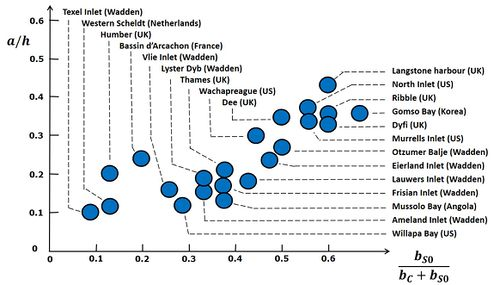Figure 4: The relative tidal amplitude $a/h$ versus the relative intertidal area $b_{S0}/(b_C+b_{S0})$ for a large number of tidal basins with small (or without) river inflow. Many of these basins (but not all) are back-barrier tidal basins or tidal lagoons: basins that are semi-closed by a sand barrier at the entrance. The figure shows a positive correlation between $a/h$ and $b_{S0}/(b_C+b_{S0})$. The correlation between these basin characteristics also depends on other parameters, in particular the tidal asymmetry already existing at the basin entrance, which is different for each basin. Therefore, one should not expect that all the point lie on a single line. Adapted from.

Because the transported sediment load increases more than linearly with the current velocity (see the articles Sand transport and Sediment transport formulas for the coastal environment), sediment fluxes during flood tide are higher than sediment fluxes during ebb tide. Flood-dominant tidal asymmetry thus produces a net import of sediment into the basin. Sediment infill could possibly go on until no tidal basin is left. This may have happened in the past to some tidal basins, but still many tidal basins without river inflow still survive. The reason is that flood dominance is neutralized by several processes. One of these processes is wave action, which can suspend large amounts of sediment in the HW period that are subsequently transported out of the basin by ebb currents. However, tidal basins do not depend only on wave action for their survival. One reason is the so-called Stokes transport, the water outflow compensating for the net influx due to greater mean water depth during flood than during ebb. Another reason is the reduction of tidal asymmetry due to the presence of intertidal areas as discussed in the previous section. During the development of intertidal areas by flood-dominant sediment transport, tidal asymmetry is weakened until the average sediment transport by flood currents has become comparable to the average transport by ebb currents. In order to neutralize flood dominance with increasing relative tidal amplitude $a/h$, the counteracting effect of intertidal areas should also increase. Fig. 4 shows that this is indeed the case for natural tidal basins with small (or without) river inflow: tidal basins with larger relative tidal amplitude have larger intertidal areas. One may thus conclude that self-organizing processes can produce a natural equilibrium morphology for tidal basins in a sedimentary environment without geological constraints  (see also the article Morphology of estuaries).

## Tidal wave deformation in a converging channel

The influence of friction on tidal propagation increases with decreasing depth. The LW propagation is slowed down more strongly than the HW propagation, which results in a larger tidal asymmetry. However, the tidal amplitude is decreased by frictional damping. During the past century many estuaries have been deepened for navigational purposes and intertidal areas have been reclaimed. The effect of these interventions on tidal propagation is illustrated in Fig. 5 for the Seine estuary and tidal river system. The tide propagates now much faster into the estuary and the tidal amplitude is much larger, especially in the upstream river. The tide propagation speed has increased more for the low waters than for the high waters, although the propagation of the high waters also benefits of the reduction of the intertidal areas. In the past a high tidal bore developed each spring tide in the downstream river. After the interventions (especially the dredging of the mouth bar) it takes a much larger distance before the HW wave crest overtakes the LW wave trough. A small tidal bore now develops far upstream and only for very high tidal coefficients.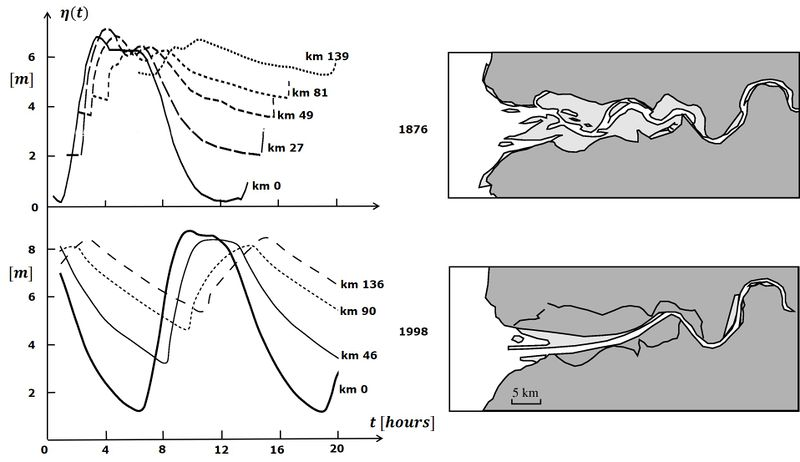Figure 5: Tidal wave distortion in the Seine estuary. The figures at the left show simultaneously recorded tide curves during springtide, for locations at various distances from the estuarine mouth. The images on the right show the morphology of the Seine estuary. The upper figures relate to the situation in the 19th century, when the morphology of the Seine estuary was almost in a natural state, with large shoals at the mouth (mouth bars) and an inner system with multiple channels and extensive intertidal areas. The 1876 tide curves (Comoy, 1881 ) display strong damping and delay in tidal propagation, especially for the low-waters. Tidal propagation over this complex shallow geometry resulted in a tidal bore that reached its largest amplitude at about 50 km from the mouth. The lower panels relate to the current situation. In the course of the 20th century, and especially in the period 1970-1980, the morphology of the estuary was greatly changed by artificial interventions. The estuarine main channel was deepened, especially in the mouth zone, and fixed by submerged dikes. Large parts of the intertidal areas were diked and filled with dredged materials. Tidal damping and tidal distortion were greatly reduced. At present a small tidal bore occurs only under extreme tides and further inland than in the past.

As discussed before, the expansion of the tidal flood wave over large intertidal areas decreases its height and propagation speed. The opposite occurs when the tide propagates into a tidal channel that becomes progressively narrower in up-channel direction, see Fig. 6. Instead of expanding laterally, the tidal wave is contracted when propagating. In the hypothetical case of no friction, conservation of the tidal energy flux along the channel requires up-channel amplification of the tidal amplitude. Many estuaries with significant river inflow have an upstream converging channel. Intertidal areas are rather small, partly as a result of natural sedimentation but often also as a result of human reclamation. The channel depth along the thalweg is fairly uniform, but shoals may be present in the mouth zone. The uniformity of the depth can also be due to dredging works for navigation purposes. In the following we consider an idealized estuary with exponentially converging width $b=b_0 \; e^{-x/L_b}$ and uniform depth $h$. It should be borne in mind that although many estuaries have an upstream converging width, the assumption of exponential width convergence and uniform depth is for most estuaries a very rough approximation. Often only a limited part of the estuary can be represented in this way.

The linearized tidal equations (cross-sectionally averaged) for this idealized estuary read:

$\Large\frac{\partial u}{\partial t}\normalsize + g \Large \frac{\partial \zeta}{\partial x}\normalsize + r \Large \frac{u}{h}\normalsize = 0 , \quad \quad (7)$

$b \Large \frac{\partial \zeta}{\partial t}\normalsize + \Large \frac{\partial (bhu)}{\partial x}\normalsize = b [ \Large \frac{\partial \zeta}{\partial t}\normalsize + h \Large \frac{\partial u}{\partial x}\normalsize – u \Large \frac{h}{L_b} \normalsize ] = 0 . \quad \quad (8)$

Solving these linear equations (only tide, no river discharge) yields

$\zeta = a e^{-\mu x} \; cos(kx-\omega t) \quad$ with $\quad 2 L_b \; \mu = \normalsize -1 + \Large[\normalsize 1 – (\Large \frac{1}{2} \normalsize + 2 K_0^2) +\large[\normalsize (\Large \frac{1}{2}\normalsize + 2 K_0^2 )^2+ 4 (K_c^2 - K_0^2) \large]^{\large 1/2} \Large]^{\large 1/2} \normalsize, \quad \quad (9)$

where $K_0 = \Large \frac{\omega L_b}{\sqrt{gh}}\normalsize , \; K_c = \Large \frac{r \omega L_b^2}{g h^2} \normalsize$. The damping factor $\mu$ is positive for large friction and large convergence length ($K_c \gt K_0$). However, for small friction and small convergence length ($K_c \lt K_0$) the damping factor is negative: the tide is amplified when propagating up-channel. Even in the case of strong friction, the tide is only slightly damped or even amplified if the convergence length $L_b$ is sufficiently small. In cases where tidal damping dominates over the effect of channel convergence (large $L_b$), the relative tidal amplitude decreases along the estuary; tidal asymmetry then becomes less relevant for upstream sediment transport. As noted before, the friction factor $r$ can vary greatly between estuaries because of salinity stratification and the type of bed sediments (coarse or muddy).

The solution of the Eqs. (7) and (8) also yields an expression for the wave propagation velocity $c$:

$c = \Large \frac{\omega}{k}\normalsize = 2 \omega L_b \Large[\normalsize - 1 + (\Large \frac{1}{2} \normalsize + 2 K_0^2) +\large[\normalsize (\Large \frac{1}{2}\normalsize + 2 K_0^2 )^2+ 4 [K_c^2 - K_0^2] \large]^{\large 1/2} \Large]^{\large -1/2}\normalsize . \quad \quad (10)$

The expressions (9) and (10) show that tidal wave propagation depends on only two parameters, $K_0$ and $K_c$. The linear equations (7) and (8) do not describe tidal wave deformation; for this, nonlinear terms have to be included ($u \partial u /\partial x$ and time varying water depth $h+\zeta$ in Eq. (7) and $b \zeta \partial u / \partial x$ in Eq. (8)). For weak nonlinearity these equations can be solved analytically ; the solution involves the additional small parameter $a/h$. Tidal wave deformation in an exponentially convergent estuary with uniform depth is entirely determined by three parameters: $K_0, K_c, a/h$ . If intertidal areas $b_S$ and mean river discharge $Q_R$ are included, two additional parameters $b_{S0}/b_C$ and $Q_R/(a b_C L_b \omega)$ are needed to characterize tidal wave deformation.

In many estuaries the nondimensional wave numbers $K_0$ and $K_c$ have similar order of magnitude. In this case $\mu \approx 0$: the longitudinal variation of the tidal amplitude is small; tidal damping through friction is offset by tidal amplification through funneling. This simpler situation was analyzed by Friedrichs and Aubrey (1994) , for converging estuaries with small relative tidal amplitudes ($a/h \lt \lt 1$) and small intertidal areas ($b_{S0}\lt \lt b_C$, Fig. 3). For the propagation speed of the HW wave crest $c_+$ and the LW wave through $c_-$ the result is

$c^{\pm} = \Large \frac{\omega}{k} \normalsize (1 \pm \Large \frac{2a}{h}\normalsize \mp \Large\frac{b_{S0}}{b_C} \normalsize) . \quad \quad (11)$

This expression shows that, as in the previous cases, the period of rising tide is shortened and the period of falling tide lengthened when the relative tidal amplitude $a/h \lt \lt 1$ increases; the tidal wave front is steepened. The opposite effect occurs when the relative width of the intertidal area $b_{S0}\lt \lt b_C$ increases.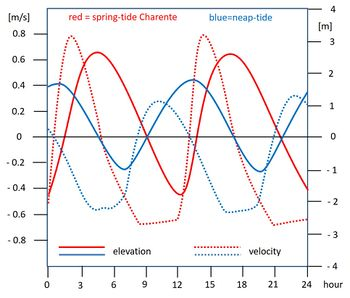Figure 7: Tidal elevation (solid) and current velocity (dotted) curves in the Charente estuary for springtide (red) and neap tide (blue). The spring tidal curves exhibit a steep tidal rise and flood currents that are stronger than ebb currents. Hardly any tidal asymmetry occurs during neap tide and ebb currents are stronger than flood currents. Data from Toublanc et al. (2015) . Characteristic parameters for the Charente estuary are: channel depth $h \approx$ 6.5 m, convergence length $L_b \approx$ 10 km.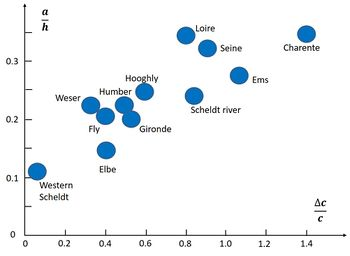Figure 8: The relative tidal amplitude $a/h$ and corresponding relative difference between HW and LW propagation speed $\Delta c / c$ for different estuaries, derived from tide gauge stations in the converging part of the estuary. Adapted from.

A large positive difference between the HW and LW propagation speeds requires a large relative tidal amplitude $a/h$. This is illustrated in Fig. 7 for the Charente estuary, by comparing the curves for tidal elevation and current velocity for springtide (large $a/h$) and neap tide (small $a/h$). During springtide the tidal rise is much steeper than for neap tide. The maximum flood current velocity is larger than the maximum ebb tidal velocity for springtide, while the opposite holds for neap tide. In Fig. 8 the relative difference between HW and LW propagation speeds $\Delta c / c = 2(c^+ -c^-)/(c^++c^-)$ are compared for estuaries with different relative tidal amplitude $a/h$. The figure shows a positive correlation between $\Delta c / c$ and $a/h$. Although $a/h$ is the most important parameter, other factors also influence the relation between $a/h$ and $\Delta c / c$, such as $K_0$ and $K_c$ (representing depth $h$, convergence length $L_b$ and friction parameter $r$), the relative intertidal area $b_{S0}/b_C$ and the mean river discharge $Q_R$. The dependence of $\Delta c / c$ on $a/h$ is therefore different for each estuary.

## Morphology of estuaries with tidal rivers

In a converging (funnel-shaped) estuary with strong friction the tidal velocity $u$ is mainly determined by the water surface slope $\partial \zeta /\partial x$, according to Eq. 4. A short period of tidal rise compared to the period of tidal fall implies steeper water surface slopes during flood than during ebb, with maximum flow rates that are higher during flood than during ebb, as illustrated in Fig. 7. Therefore, sediment fluxes during flood tide are higher than sediment fluxes during ebb tide, resulting in a net import of sediment into the estuary. As shown before, flood dominance increases with increasing relative tidal amplitude $a/h$. The time span over which flood dominance develops also plays a role. For converging estuaries the relevant nondimensional time duration indicator is mainly determined by the ratio of convergence length and tide propagation speed $L_b \omega/ c$. The value of the nondimensional parameter $a L_b \omega / (h c)$ can be considered an indication for the strength of flood dominance.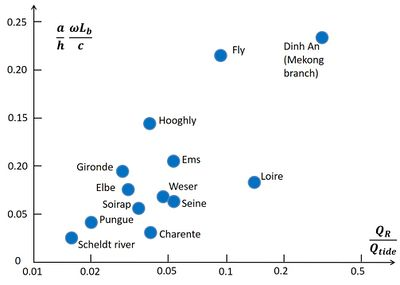Figure 9: Tidal asymmetry indicator versus river flow indicator for different estuaries. A positive correlation is an indication that river flow makes an important contribution to compensating for tide-induced sediment import. The spread in the data is related to many other factors that influence sediment import/export. Adapted from .

Infill of estuaries is limited by sediment export through river flow, although dredging may also play a role. The influence of river flow on sediment export can be represented by the nondimensional parameter $Q_R/Q_{tide}$, where $Q_R$ is the mean river discharge and $Q_{tide}$ the maximum tidal discharge in the mid-estuarine zone ($x \approx L_b/2$). For estuaries in morphological equilibrium, sediment import due to tidal asymmetry (flood dominance) should be approximately balanced by export due to river flow. Comparing different estuaries one may thus expect a positive correlation between the parameters $a L_b \omega / (h c)$ and $Q_R/Q_{tide}$ . As shown in Fig. 9, such a positive correlation exists, although the spread in the data is large. This spread can be due to many other factors, which influence sediment import and export in different ways. Possible important factors are:

• dredging,
• import/export by wave activity,
• import by estuarine circulation,
• sediment recirculation in ebb/flood-channel cells,
• sediment import/export related to settling and erosion time lags,
• fluvial sediment supply,
• type of sediment.

## Related articles

Morphology of estuaries
Ocean and shelf tides
Tidal bore dynamics
Tidal motion in shelf seas
Estuarine circulation